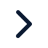Executive ProgramsWorkshopsProjectsBlogsCareersFind JobsCorporate TrainingHire from USAll Courses

Choose a category

All Courses

All CoursesHyperelastic material modelling and validation using LS-DYNA

Understand how to perform a tensile test to calculate the Mooney Rivlin and Ogden material parameters and compare it with the given stress-strain data. Learn how to use hyper-elastic material card and give particular inputs for simulation. Study and understand conversions from engineering stress-strain to true stress-strain and terms like stretch ratio and extension. Finally, understand curve fitting and compare the simulation and given stress-strain data.

• Mechanical/Automotive/Material scienceDuration :

1 monthProject Fees :

INR 30,000

Benefits of this Project

Understand how to perform a tensile test to calculate the Mooney Rivlin and Ogden material parameters and compare it with the given stress-strain data. Learn how to use hyper-elastic material card and give particular inputs for simulation. Study and understand conversions from engineering stress-strain to true stress-strain and terms like stretch ratio and extension. Finally, understand curve fitting and compare the simulation and given stress-strain data.

What will you do in this project?Step 1 - Applying boundary conditions to the given geometryStep 2 - Curve fitting for different hyper-elastic material constantsStep 3 - Post-processing in LS-PrePostStep 4 - Validation of hyper-elastic material model

You will be performing a tensile test on a dogbone specimen to validate the hyper-elastic material model. You will do the following in this project,

• Input experimental stress-strain data through hyper-elastic material card
• Set-up a tensile test case study by applying required boundary conditions in LS-PrePost
• Evaluate the fitted curves for various number of constants for the hyper-elastic material model
• Tensile test simulation using the obtained constants and result extraction
• Analyse and compare the stress-strain results with the given data

Similar Projects you might be interested:Elasto-plastic material modelling and validation using LS-DYNA
In this project, you will learn how to validate the elasto-plastic material model with the given stress-strain data. You will learn the extraction and cleaning of the stress-strain data point from the given curve. Then you will study how to evaluate the hardening curve and give material parameters input to the LS-DYNA software. Finally, you will understand how to extract the stress-strain results from the simulation and compare it with the given data.Duration:

1 monthDomain :

Mechanical/Automotive/Material science/Metal formingIn this project, you will understand modeling of Material LAW-1- Elastic, LAW-2, LAW36 - Elastoplastic, and LAW-27-Brittle along with Johnson-Cook failure in Radioss. You will also understand XFEM formulation to understand the effect of cracking in the model.Duration:

1 monthDomain :

Mechanical Engineering , Automotive Engineering , Materials Engineering

Showing 1 of 2 projects

Project Highlights

The project is an advanced level project

Pre-requisites

Work with Multiple ToolsHyper-elastic material modelling and validation using LS-DYNA

In this project, you will simulate a tensile test on a given dogbone specimen to validate the hyper-elastic material model in LS-DYNA. The experimental stress-strain data is given and is used as an input which defines the uniaxial stress-strain behaviour. understand how to give all boundary conditions for a tensile test and set up an error-free input file for the solver. Finally, run the simulation using this input file using the launch manager. Understand conversions from engineering stress-strain curve to true stress-strain curve and terms like stretch/extension. Post-processing would be carried out in LS-PrePost and simulated results would be compared to validate the material model.

Try our top engineering courses, projects & workshops today!